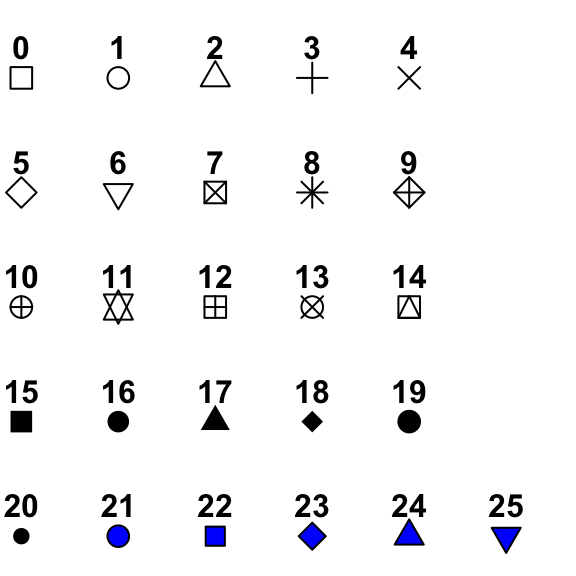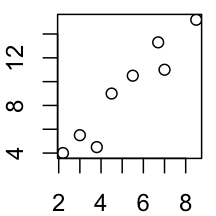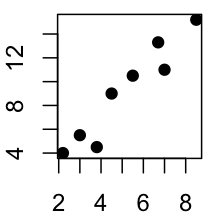# R plot pch symbols : The different point shapes available in R

Different plotting symbols are available in R. The graphical argument used to specify point shapes is pch.

# Plotting symbols

The different points symbols commonly used in R are shown in the figure below :The function used to generate this figure is provided at the end of this document

• pch = 0,square
• pch = 1,circle
• pch = 2,triangle point up
• pch = 3,plus
• pch = 4,cross
• pch = 5,diamond
• pch = 6,triangle point down
• pch = 7,square cross
• pch = 8,star
• pch = 9,diamond plus
• pch = 10,circle plus
• pch = 11,triangles up and down
• pch = 12,square plus
• pch = 13,circle cross
• pch = 14,square and triangle down
• pch = 15, filled square
• pch = 16, filled circle
• pch = 17, filled triangle point-up
• pch = 18, filled diamond
• pch = 19, solid circle
• pch = 20,bullet (smaller circle)
• pch = 21, filled circle blue
• pch = 22, filled square blue
• pch = 23, filled diamond blue
• pch = 24, filled triangle point-up blue
• pch = 25, filled triangle point down blue

Point can be omitted from the plot using pch = NA.

# Examples

``````x<-c(2.2, 3, 3.8, 4.5, 7, 8.5, 6.7, 5.5)
y<-c(4, 5.5, 4.5, 9, 11, 15.2, 13.3, 10.5)
# Plot points
plot(x, y)
# Change plotting symbol
# Use solid circle
plot(x, y, pch = 19)``````By default pch=1

The following arguments can be used to change the color and the size of the points :

• col : color (code or name) to use for the points
• bg : the background (or fill) color for the open plot symbols. It can be used only when pch = 21:25.
• cex : the size of pch symbols
• lwd : the line width for the plotting symbols
``````# Change color
plot(x, y, pch=19, col="darkgreen", cex=1.5)
# Color can be a vector
plot(x, y, pch=19, col=c("green", "red"))
# change border, background color and line width
plot(x, y, pch = 24, cex=2, col="blue", bg="red", lwd=2)``````Note that, only pch from 21 to 25 can be filled using the argument bg=

Other characters can be used to specify pch including “+”, “*“,”-“,”.“,”#, “%”, “o”

``````set.seed(1234)
plot(x=rnorm(100), y=rnorm(100), pch="+")``````# R script to generate a plot of point shapes in R

Use the following R function to display a graph of the plotting symbols :

``````#++++++++++++++++++++++++++++++++++++++++++++
#generate a plot of point shapes which R knows about.
#++++++++++++++++++++++++++++++++++++++++++++
generateRPointShapes<-function(){
oldPar<-par()
par(font=2, mar=c(0.5,0,0,0))
y=rev(c(rep(1,6),rep(2,5), rep(3,5), rep(4,5), rep(5,5)))
x=c(rep(1:5,5),6)
plot(x, y, pch = 0:25, cex=1.5, ylim=c(1,5.5), xlim=c(1,6.5),
axes=FALSE, xlab="", ylab="", bg="blue")
text(x, y, labels=0:25, pos=3)
par(mar=oldPar\$mar,font=oldPar\$font )
}
generateRPointShapes()``````

# Infos

``This analysis has been performed using R (ver. 3.1.0).``